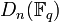# Chevalley group of type D

Jump to: navigation, search

## Definition

Let$K$ be a field and$n$ be a natural number. The Chevalley group of type D denoted$D_n(K)$, is defined as follows:

1. Start with the split orthogonal group of degree$2n$ over$K$.
2. Consider the intersection of the kernel of the spinor norm map with the kernel of the Dickson invariant map (note that the kernel of the Dickson invariant map is the same as the split special orthogonal group, i.e., the kernel of the determinant, when the characteristic is not 2)
3. Consider the inner automorphism group of this intersection, i.e., quotient it out by its center.

The final answer obtained in Step (3) is denoted$D_n(K)$. It is also denoted$P\Omega_{2n}^+(K)$.

The notation$D_n(q)$ is used as a shorthand for$D_n(\mathbb{F}_q)$ where$\mathbb{F}_q$ is the (unique up to isomorphism) field of size$q$.

## Particular cases

### Finite fields

PLACEHOLDER FOR INFORMATION TO BE FILLED IN: [SHOW MORE]$q$ (field size)$p$ (underlying prime, field characteristic) exponent on$p$ giving$q$$n$ Group$D_n(q)$ Order